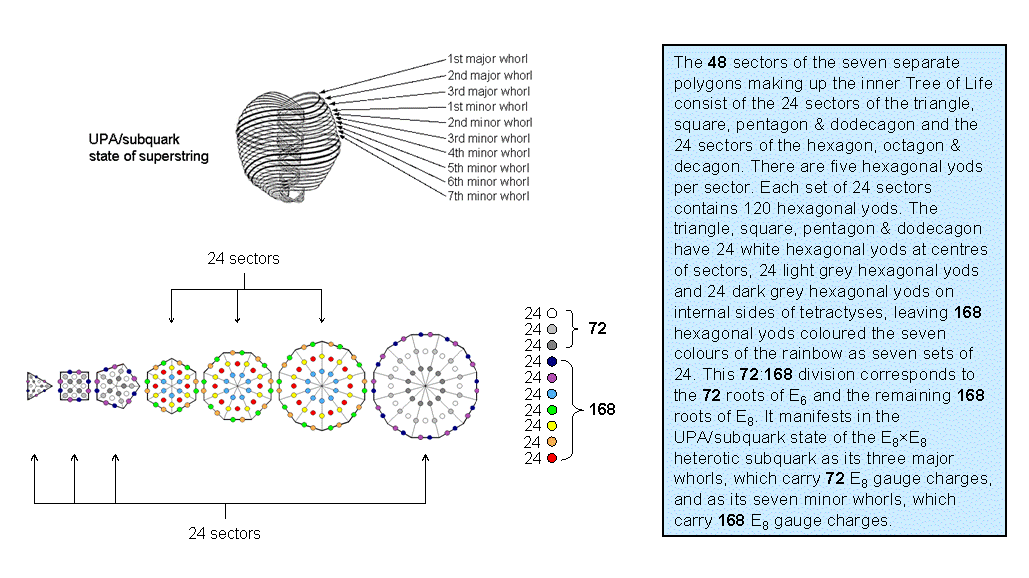<< Previous    1...   31  32    34  35  ...50    Next >>

The 10-fold UPA/superstring as the 10-fold division in the 240 hexagonal yods in the seven separate polygons of the inner Tree of LifeThe seven separate polygons of the inner Tree of Life have 48 sectors, where 48 is the number value of Kokab, the Mundane Chakra of Hod. As tetractyses, they contain 240 hexagonal yods that divide into ten sets of 24, as now explained.

As a parameter of holistic systems, the number 48 displays a 24:24 division that is characteristic of them. For the seven polygons, this is the triangle, square, pentagon & dodecagon, which have 24 sectors, and the hexagon, octagon & decagon, which, too, have 24 sectors. The only other possible combinations of polygons with 24 sectors are firstly, the triangle, pentagon, hexagon & decagon, and secondly, the square, octagon & dodecagon (the two possibilities arise because the triangle and pentagon have the same number of sectors as the octagon). Just as the seven Sephiroth of Construction comprise two triads: Chesed-Geburah-Tiphareth and Netzach-Hod-Yesod, and Malkuth, so research by the author has confirmed that this 3:3:1 pattern exists for the seven polygons of the inner Tree of Life, i.e, they group into triangle-square-pentagon, hexagon-octagon-decagon & dodecagon. Only the former combinations of polygons respect this grouping because the latter combinations are each taken from both sets of three polygons. Although, arithmetically speaking, either pair of combinations is possible because each combination has 24 corners, the former will be considered here because it preserves the analogy with the Tree of Life. There are five hexagonal yods per sector. Therefore, the 240 hexagonal yods comprise 24 sets of five in the triangle, square, pentagon & dodecagon and 24 sets of five in the hexagon, octagon & decagon. Alternatively, they may be regarded as five sets of 24 and another five sets of 24, i.e., as ten sets of 24. This 5:5 differentiation reflects the division of the Tree of Life into the so-called "Upper Face" spanned by the highest five Sephiroth and the "Lower Face" spanned by the lowest five Sephiroth. It arises here from the primary division of the 48 sectors into two sets of 24.

The diagram displays the ten sets of 24 hexagonal yods, the yods in each set having the same colour. Each colour corresponds to one of the ten Sephirah. The white, light grey & dark grey yods correspond to the Supernal Triad, which, being part of the Upper Face, means that these yods belong to the triangle, square, pentagon & dodecagon. The question is: which of the five hexagonal yods in each sector should the white, light grey & dark grey hexagonal yods be? For overlapping Trees of Life, Kether, Chokmah & Binah of a given Tree become Tiphareth, Netzach & Hod of the next higher Tree, whilst Tiphareth is Malkuth of the next higher Tree, symbolized by the hexagonal yod at the centre of a tetractys. These Sephiroth of Construction belong to different triads. Hence, the white, light grey and dark grey hexagonal yods are the central hexagonal yod and the two hexagonal yods either on a side of a polygon or on an internal side of a tetractys. The latter possibility has been chosen here.

The ten sets of 24 hexagonal yods symbolize the ten whorls of the UPA/subquark state of the E8×E8 heterotic superstring. The white hexagonal yods at the centres of the 24 sectors in the triangle, square, pentagon & dodecagon denote the 24 E8 gauge charges carried by the first major whorl, the light grey hexagonal yods denote the 24 E8 gauge charges carried by the second major whorl, etc. The ten-fold pattern of the distribution of hexagonal yods arises from the facts that there are five such yods per sector and that the 48 sectors of the seven polygons divide into two sets of 24.

 << Previous    1...   31  32    34  35  ...50    Next >>

Home